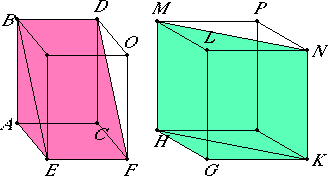# Proposition 39

If there are two prisms of equal height, and one has a parallelogram as base and the other a triangle, and if the parallelogram is double the triangle, then the prisms are equal.

Let ABCDEF and GHKLMN be two prisms of equal height, let one have the parallelogram AF as base, and the other the triangle GHK, and let the parallelogram AF be double the triangle GHK.

I say that the prism ABCDEF equals the prism GHKLMN.Complete the solids AO and GP.

I.34

Since the parallelogram AF is double the triangle GHK, and the parallelogram HK is also double the triangle GHK, therefore the parallelogram AF equals the parallelogram HK.

XI.31

But parallelepipedal solids on equal bases of the same height equal one another, therefore the solid AO equals the solid GP.

XI.28

And the prism ABCDEF is half of the solid AO, and the prism GHKLMN is half of the solid GP, therefore the prism ABCDEF equals the prism GMKLMN.

Therefore, if there are two prisms of equal height, and one has a parallelogram as base and the other a triangle, and if the parallelogram is double the triangle, then the prisms are equal.

Q.E.D.

## Guide

This proposition is designed specifically to take care of a situation that occurs in propositions XII.3 and XII.4 on the way to proving XII.5 concerning the volume of a pyramid.

Both of the prisms in this proposition are triangular, but the base of the first is taken to be one of the parallelograms ACFE on its side while the base of the second is a triangular end GHK. To say that they have the height means the distance from the vertex B to the plane of the parallelogram ACEF is the same as the distance from the vertex M to the plane of the triangle GHK.

When the solids are completed, they are doubled to create two parallelepipeds of the same height and equal bases, which therefore are equal, and so are their halves, the original prisms.